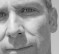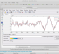## The first algorithm for computing the inverse chirp z-transform (ICZT) in O(n log n) timeStarted by 4 years ago3 replieslatest reply 4 years ago811 views

Big news in the DSP world; a couple of researchers from Iowa State University have come up with an algorithm to compute the inverse chirp z-transform (ICZT) in O(n log n) time.

I created this thread because I think the DSP community could benefit from a discussion on how significant this new development is, what are and will be the implications, the practical applications, etc.

Thanks in advance for any insight you may contribute to this discussion.

Stephane

[ - ]To put this paper into perspective, multiplying a general N^2 matrix by an N-dimensional vector requires N^2 multiplications (N times a row of the matrix times the vector which is itself N multiplications).

The Cooley-Tukey FFT reduces this to N log N by exploiting the fact that the matrix W has a very special structure - powers of the Nth root of unity. So W can be written as the product of a permutation matrix (bit reversal) and log N matrices, each of which only performs N multiplications on the vector.

The fast CZT uses an alternative which could have been used for the FFT as well. Here the W matrix is written as the product of a diagonal matrix, a Toeplitz matrix, and another diagonal matrix. These diagonal matrices, and the chirp diagonal matrix, each obviously perform N multiplications on the vector. It is well-known that multiplying a Toeplitz matrix by a vector can be done in O(N log N), so that is the dominant contribution.

Performing the iCZT requires multiplication of the inverse of the CZT matrix by a vector. The authors start with the CZT matrix decomposition as just described, and (by extending a known theorem) show that the inverse of a symmetric Toeplitz matrix can be written as 1/u0 (A At - U Ut) where A is a Toeplitz matrix with main diagonal and below (and At is its transpose), U is an upper triangle Toeplitz matrix (and Lt is its transpose) and u0 is a scalar which happens to be the element on the main diagonal of A.

Once again the full product has diagonal matrices (which contribute O(N)) and Toeplitz matrices (which contribute O(N log N).

Y(J)S

[ - ]Hi. That inverse chirp-z transform (ICZT) algorithm looks interesting, and I complement the Iowa State University professors for their clever work. However I can't think of any applications for the ICZT except for, perhaps, generating time-domain "test" signals that have a specialized spectral nature.

By the way, the professors claim the computational time of the standard forward chirp z-transform is O(n log n). I've always thought the computational time of the forward chirp z-transform is O((n+m) log (n+m)) where 'n' is the number of input time samples and 'm' is the number of computed spectral samples.

[ - ]